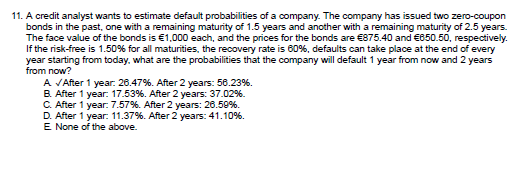# Probability of default for bonds

Hello everyone,

Currently I am trying to solve a question regarding default probabilities.Where I do
Coupon 1 value: 1000exp(-0,00753)=977,75
Coupon 2 value 1000* exp(-0,0075*5)=963,19

Exp Loss Coupon 1 = 977,75-875,4=102,35
Exp loss coupon 2 = 963,19-650,5 = 312,69

PD1:
PV of LGD (40%1000)= 391,1 (400exp(-0,0075*3)
PD 1 = 102,35/391,1=26,17%

And for PD 2 I am completely unaware of what has to be done

Can anyone help me with this?

Kind regards,
Luc

I’m just wondering why you are assuming continuously compounded interest. Also, I treated the 600 as coming in 1 year from now and the full 1,000 arriving at time 1.5. If I use 1.5% effective annual, I get a 1 year default probability of 0.2650.

ETA: For year 2, I think you have to do something similar to year 1. Equate the market price to the expected payouts, but the wrinkle here is that you have to add an extra term for the bond “surviving” to the start of year 2 but defaulting at the end of year 2.

Thanks for the help, but I still dont get how exactly you compute this. I just keep ending up with 26,17%. Could you show a calculation please?

My approach may be slightly different that what your texts are telling you. Also, I am using effective annual compounding and recoveries happen at time of default.

Let D represent the 1-year default rate
875.4 = D * 600*(1.015)^-1 + (1 - D) * 1,000 * (1.015)^-1.5
875.4 = 586.748 * D + (1 - D) * 977.446
-102.51 = - 386.781 D
D = 0.26503